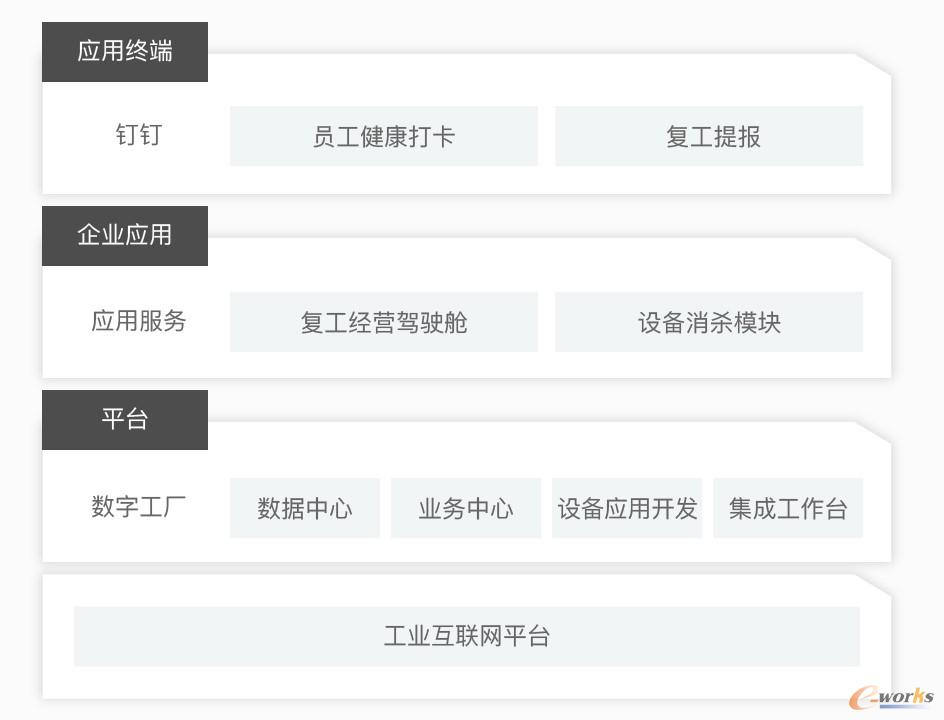+关注继续查看

什么是快速排序

快速排序简介快速排序的分治思想如何划分

Partition(A,p,q)   // A[p,..q]
1   x=A[p]   // pivot=A[p] 主元
2   i=p
3   for j=p+1 to q
4       do if A[j]<=x
5          then i=i+1
6             exch A[i]<->A[j]
7   exch A[p]<->A[i]
8   return i // i pivot将递归的思想作用于划分上

Quicksort(A,p,q)
1   if p<q
2     then r=Partition(A,p,q)
3          Quicksort(A,p,r-1)
4          Quicksort(A,r+1,q)

快速排序的算法分析

怎样是最坏的划分

1）输入的元素已经排序或逆向排序
2）每个划分的一边都没有元素

T(n)=T(0)+T(n1)+\Theta(n)=Θ(1)+T(n1)+Θ(n)=Θ(n1)+Θ(n)=Θ(n2)T(n)=Θ(n)+Θ(n2)=Θ(n2)

最坏划分的算法分析

T(n)=max0rn1(T(r)+T(nr1))+Θ(n)

T(n)max0rn1(cr2+c(nr1)2)+Θ(n)cmax0rn1(r2+(nr1)2)+Θ(n)

1）而r2+(nr1)2对于r的二阶导数为正，所以在区间0rn1的右端点取得最大值。

T(n)cn2c(2n1)+Θ(n)

2）r2+(nr1)2对于r的二阶导数为正，所以在区间0rn1的左端点取得最小值。

T(n)cn2c(2n1)+Θ(n)

怎样是最好的划分

T(n)=2T(n/2)+Θ(n)=Θ(nlgn)

怎样是平衡的划分

T(n)=T(110n)+T(910n)+Θ(n)T(n)cnlog109n+Θ(n)

T(n)=Θ(nlgn)

随机化快速排序

随机算法的思想

【算法】3 由招聘问题看随机算法中我们介绍了随机算法，它使得对于所有的输入都有着较好的期望性能，因此随机化快速排序在有大量数据输入的情况下是一种更好的排序算法。

1）其运行时间不依赖与输入序列的顺序

2）无需对输入序列的分布做任何假设

3）没有 一种特别的输入会引起最差的运行情况

4）最差的情况由随机数产生器决定

随机抽样技术

RANDOMIZED-PARTITION(A,p,q)
1   i=RANDOM(p,q)
2   exchange A[p] with A[i]
3   return PARTITION(A,p,q)
RANDOMIZED-QUICKSORT(A,p,q)
1   if p<q
2       r=RANDOMIZED-PARTITION(A,p,q)
3       RANDOMIZED-QUICKSORT(A,p,r-1)
4       RANDOMIZED-QUICKSORT(A,r+1,q)

回顾比较排序

插入排序选择排序归并排序堆排序快速排序另外一些比较排序

冒泡排序奇偶排序template <class T>
void OddEvenSort (T a[], int n)
{
for (int i = 0 ; i < n ; i++)
{
if (i & 1) // 'i' is odd
{
for (int j = 2 ; j < n ; j += 2)
{
if (a[j] < a[j-1])
swap (a[j-1], a[j]) ;
}
}
else
{
for (int j = 1 ; j < n ; j += 2)
{
if (a[j] < a[j-1])
swap (a[j-1], a[j]) ;
}
}
}
}

双向冒泡排序排序算法的下界

决策树n!l2h

=> lg2hlgn!

=> lg2h=hlg2lgn!

lg2<1

nlgn!=Ω(nlgn)

线性时间排序

计数排序

COUNTING-SORT(A,B,k)
1   let C[0...k] be a new array
2   for i=0 to k
3       C[i]=o
4   for j=1 to A.length
5       C[A[j]]=C[A[j]]+1
6   // C[i] now contains the number of element equal to i.
7   for i=1 to k
8       C[i]=C[i]+C[i-1]
9   // C[i] now contains the number of element less than or equal to i.
10  for j=A.length downto 1
11      B[C[A[j]]]=A[j]
12      C[A[j]]=C[A[j]]-1k=O(n)时，计数排序的运行时间为Θ(n)

基数排序桶排序

BUCKET-SORT(A)
1   n=A.length
2   let B[0...n-1] be a new array
3   for i=0 to n-1
4       make B[i] an empty list
5   for i=1 to n
6       insert A[i] into list B[小于等于nA[i]的最大整数]
7   for i=0 to n-1
8       sort list B[i] with insertion sort
9   concatenate the lists B,B,...B[n-1] together in order9485 0Java基础-14总结正则表达式，Pattern，Mactcher，Math，BigInteger，BigDeximal，System等

2012 010842 0323 0javascript 一个关于时间排序的算法（一个页面多个倒计时排序）

852 013168 0python3中，os.path模块下常用的用法总结
abspath basename dirname exists getatime getctime getmtime getsize isabs isdir isfile islink ismount join realpath samefile sameopenfile split splitext abspath 返回一个目录的绝对路径 Return an absolute path.
777 0Java数组排序基础算法,二维数组，排序时间计算，随机数产生
import java.util.Arrays; //包含Arrays import java.util.Random; public class HelloWorld { public static void main(String[] args){ // Scanner s = new Scanner(System.
853 01522 0
542

0

《2021云上架构与运维峰会演讲合集》

《零基础CSS入门教程》

《零基础HTML入门教程》